## D. Artificial Dissipation Terms

Numerical damping terms are introduced for numerical stability.

### Acoustic damping term

The acoustic damping terms are added in the momentum equations (A.4) and (A.5) for the sake of stable numerical integration of acoustic modes .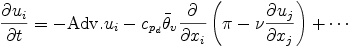where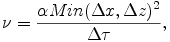Δ τ is the short time step for integration of the acoustic mode. The dumping coefficient &alpha is set to be 5 × 10-9.

### Numerical diffusion term

Numerical diffusion terms are added to all of the prognostic equations to suppress numerical noise which is generated by the centered difference scheme for advection terms.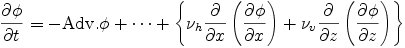where φ represents each prognostic variable, and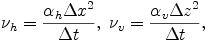Δ t is the long-time step. The dumping coefficients αh and αv are set to be 1×10-4.

### Rayleigh damping term

The Rayleigh damping term is added to the momentum equations (A.4) and (A.5) in order to absorb upward propagating wave disturbances and to prevent wave reflection at the upper boundary.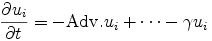where γ is given as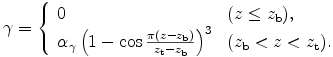Here, zb = 2.0 × 106 m, zt = 3.0 × 106 m, and αγ = 1/500 s-1.